• 通过傅立叶np.fft.fftshift()，将图像的低频部分移动到图像的中心位置。然后，通过高通滤波器覆盖掉中心低频部分，将255两点变换为0，同时保留高频部分。 # -*- coding: utf-8 -*- import cv2 as cv import numpy ...
参考
https://blog.csdn.net/Eastmount/article/details/89645301
https://www.cnblogs.com/my-love-is-python/p/10406038.html
1.cv2.dft(进行傅里叶变化)
cv2.dft(img, cv2.DFT_COMPLEX_OUTPUT) 进行傅里叶变化
参数说明: img表示输入的图片， cv2.DFT_COMPLEX_OUTPUT表示进行傅里叶变化的方法
2.np.fft.fftshift(将低频移动到图像的中心)
np.fft.fftshift(img)  将图像中的低频部分移动到图像的中心
参数说明：img表示输入的图片
3.cv2.magnitude(计算矩阵的加和平方根)
cv2.magnitude(x, y) 将sqrt(x^2 + y^2) 计算矩阵维度的平方根
参数说明：需要进行x和y平方的数
4.np.fft.ifftshift(将低频和高频移动到原来位置)
np.fft.ifftshift(img) # 进图像的低频和高频部分移动到图像原来的位置
参数说明：img表示输入的图片
5.cv2.idft(傅里叶逆变换)
cv2.idft(img) # 进行傅里叶的逆变化
参数说明：img表示经过傅里叶变化后的图片
傅里叶变化：将图像从空间域转换为频率域， 下面是傅里叶变化的公式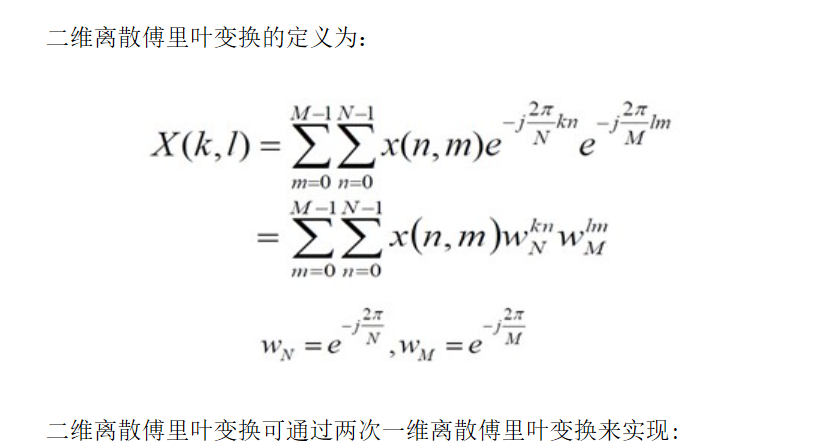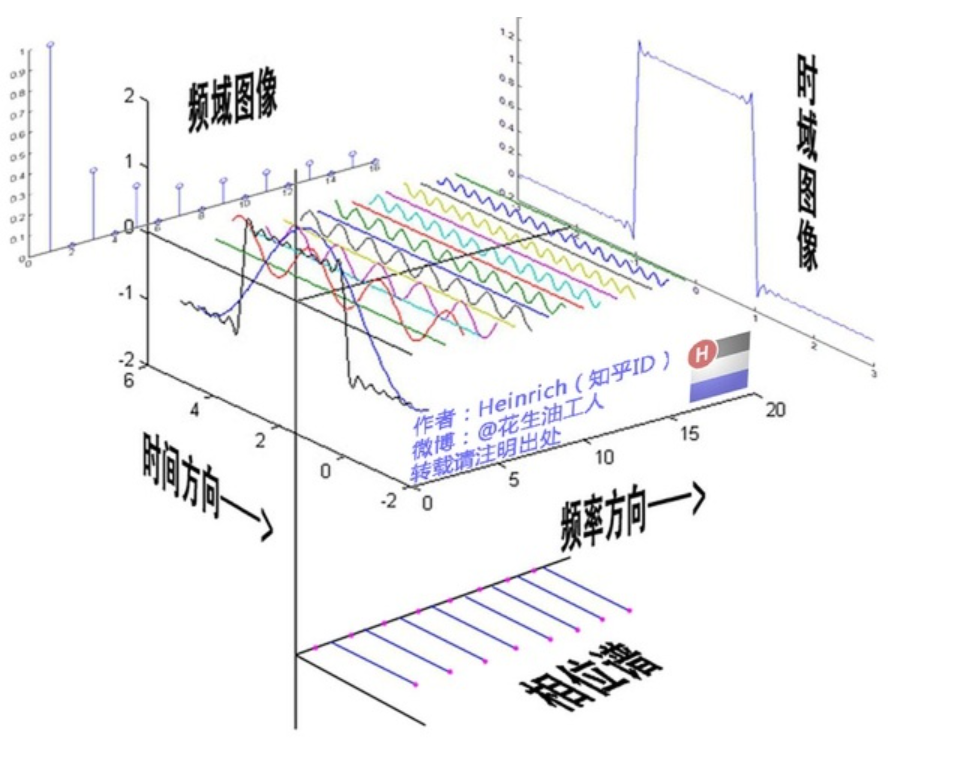对应于频率的方向，我们可以看出红色那条线的频率最小，蓝色线的频率最大， 高频指变化剧烈的灰度分量，即图像边界的地方, 低频指变换缓慢的灰度分量。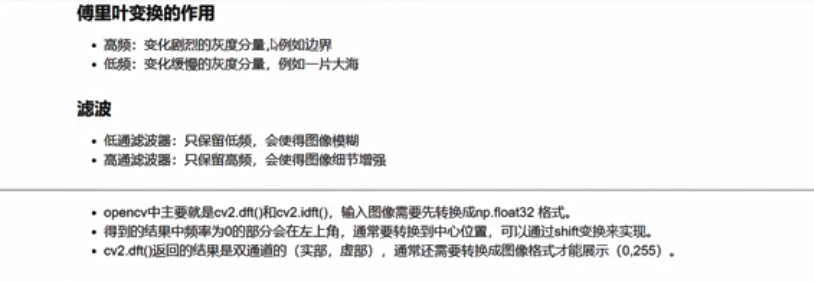构建出的傅里叶变化的图片，将低频移到中间位置， 通常呈现中间亮，周围暗，是因为对于低频而言，波动较大，比如红色那条线，因此呈现亮，对于高频而言，波动较小，比如蓝色那条线，因此呈现暗
代码：
第一步：载入图片
第二步：使用np.float32进行格式转换
第三步：使用cv2.dft进行傅里叶变化
第四步：使用np.fft.shiftfft将低频转移到中间位置
第五步：使用cv2.magnitude将实部和虚部投影到空间域
第六步：进行作图操作
# -*- coding: utf-8 -*-
import cv2
import numpy as np
import matplotlib.pyplot as plt
# 第一步读取图片
img = cv2.imread('lena.jpg', 0)
# 第二步：进行float32形式转换
float32_img = np.float32(img)
# 第三步: 使用cv2.dft进行傅里叶变化
dft_img = cv2.dft(float32_img, flags=cv2.DFT_COMPLEX_OUTPUT)
# 第四步：使用np.fft.shiftfft()将变化后的图像的低频转移到中心位置
dft_img_ce = np.fft.fftshift(dft_img)
# 第五步：使用cv2.magnitude将实部和虚部转换为实部，乘以20是为了使得结果更大
img_dft = 20 * np.log(cv2.magnitude(dft_img_ce[:, :, 0], dft_img_ce[:, :, 1]))
# 第六步：进行画图操作
plt.subplot(121)
plt.imshow(img, cmap='gray')
plt.subplot(122)
plt.imshow(img_dft, cmap='gray')
plt.show()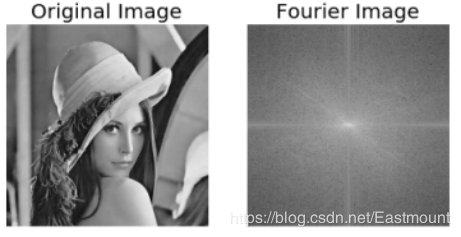滤波器
傅里叶变换的目的并不是为了观察图像的频率分布（至少不是最终目的），更多情况下是为了对频率进行过滤，通过修改频率以达到图像增强、图像去噪、边缘检测、特征提取、压缩加密等目的。
过滤的方法一般有三种：低通（Low-pass）、高通（High-pass）、带通（Band-pass）。所谓低通就是保留图像中的低频成分，过滤高频成分，可以把过滤器想象成一张渔网，想要低通过滤器，就是将高频区域的信号全部拉黑，而低频区域全部保留。
低通滤波器
只保留低频，即进行低通滤波，因为高频表示是一些细节，即图像的轮廓和边缘，失去了高频部分，图像就容易变得模糊
代码：
第一步：读取图片
第二步：np.float32进行类型转换
第三步：使用cv2.dft进行傅里叶变化
第四步：使用np.fft.fftshift 将低频部分转换到图像的中心
第五步：构造掩模，使得掩模的中心位置为1，边缘位置为0
第六步：将掩模与傅里叶变换后的图像结合，只保留中心部分的低频位置
第七步：使用np.fft.ifftshift将低频部分转移回图像的原先位置
第八步：使用cv2.idft进行傅里叶的反转换
第九步：使用cv2.magnitude将图像的实部和虚部转换为空间域内
第十步：进行作图操作
# -*- coding: utf-8 -*-
import cv2 as cv
import numpy as np
from matplotlib import pyplot as plt

# 第一步读入图片
img = cv2.imread('test.jpg', 0)
# 第二步：进行数据类型转换
img_float = np.float32(img)
# 第三步：使用cv2.dft进行傅里叶变化
dft = cv2.dft(img_float, flags=cv2.DFT_COMPLEX_OUTPUT)
# 第四步：使用np.fft.fftshift将低频转移到图像中心
dft_center = np.fft.fftshift(dft)
# 第五步：定义掩模：生成的掩模中间为1周围为0
crow, ccol = int(img.shape / 2), int(img.shape / 2) # 求得图像的中心点位置
mask = np.zeros((img.shape, img.shape, 2), np.uint8)
mask[crow-30:crow+30, ccol-30:ccol+30] = 1

# 第六步：将掩模与傅里叶变化后图像相乘，保留中间部分
mask_img = dft_center * mask

# 第七步：使用np.fft.ifftshift(将低频移动到原来的位置
img_idf = np.fft.ifftshift(mask_img)

# 第八步：使用cv2.idft进行傅里叶的反变化
img_idf = cv2.idft(img_idf)

# 第九步：使用cv2.magnitude转化为空间域内
img_idf = cv2.magnitude(img_idf[:, :, 0], img_idf[:, :, 1])

# 第十步：进行绘图操作
plt.subplot(121)
plt.imshow(img, cmap='gray')
plt.subplot(122)
plt.imshow(img_idf, cmap='gray')
plt.show()

高通滤波器
高通滤波器是指通过高频的滤波器，衰减低频而通过高频，常用于增强尖锐的细节，但会导致图像的对比度会降低。该滤波器将检测图像的某个区域，根据像素与周围像素的差值来提升像素的亮度。通过傅立叶np.fft.fftshift()，将图像的低频部分移动到图像的中心位置。然后，通过高通滤波器覆盖掉中心低频部分，将255两点变换为0，同时保留高频部分。
# -*- coding: utf-8 -*-
import cv2 as cv
import numpy as np
from matplotlib import pyplot as plt

#读取图像
img = cv.imread('test.png', 0)

#傅里叶变换
f = np.fft.fft2(img)
fshift = np.fft.fftshift(f)

#设置高通滤波器
rows, cols = img.shape
crow,ccol = int(rows/2), int(cols/2)
fshift[crow-30:crow+30, ccol-30:ccol+30] = 0

#傅里叶逆变换
ishift = np.fft.ifftshift(fshift)
iimg = np.fft.ifft2(ishift)
iimg = np.abs(iimg)

#显示原始图像和高通滤波处理图像
plt.subplot(121), plt.imshow(img, 'gray'), plt.title('Original Image')
plt.axis('off')
plt.subplot(122), plt.imshow(iimg, 'gray'), plt.title('Result Image')
plt.axis('off')
plt.show()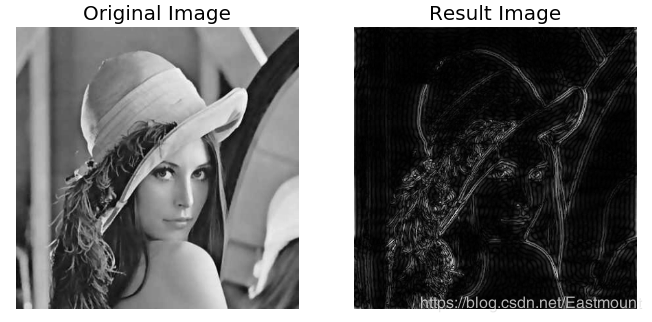另为一种写法：
# -*- coding: utf-8 -*-
import cv2
import numpy as np
import matplotlib.pyplot as plt

# 第一步读入图片
img = cv2.imread('test.jpg', 0)
# 第二步：进行数据类型转换
img_float = np.float32(img)
# 第三步：使用cv2.dft进行傅里叶变化
dft = cv2.dft(img_float, flags=cv2.DFT_COMPLEX_OUTPUT)
# 第四步：使用np.fft.fftshift将低频转移到图像中心
dft_center = np.fft.fftshift(dft)
# 第五步：定义掩模：生成的掩模中间为0周围为1
crow, ccol = int(img.shape / 2), int(img.shape / 2) # 求得图像的中心点位置
mask = np.ones((img.shape, img.shape, 2), np.uint8)
mask[crow-30:crow+30, ccol-30:ccol+30] = 0

# 第六步：将掩模与傅里叶变化后图像相乘，保留中间部分
mask_img = dft_center * mask

# 第七步：使用np.fft.ifftshift(将低频移动到原来的位置
img_idf = np.fft.ifftshift(mask_img)

# 第八步：使用cv2.idft进行傅里叶的反变化
img_idf = cv2.idft(img_idf)

# 第九步：使用cv2.magnitude转化为空间域内
img_idf = cv2.magnitude(img_idf[:, :, 0], img_idf[:, :, 1])

# 第十步：进行绘图操作
plt.subplot(121)
plt.imshow(img, cmap='gray')
plt.subplot(122)
plt.imshow(img_idf, cmap='gray')
plt.show()



展开全文• opencv实现快速傅立叶变换和逆变换


原文： http://www.tuicool.com/articles/VB3UNjf
说实话觉得网上很多人转载的文章的挺坑的，全部是opencv文档程序的翻译，看来看去都是那一
篇，真的没啥意思。
文档的地址。
本来opencv实现dft就是一个函数的事情，但是很少有关于逆变换使用的资料。我这几天在翻译
matlab版本的L0Smooth到opencv上面，就碰到这样一件很坑爹的事情。
首先，很少有人说清楚这个函数的使用方法。还有，根据教程，dft之前最好扩充原矩阵到合适的尺
寸(2,3,5的倍数)，再调用dft会加快速度。那么，idft的时候了？如何恢复原有的尺寸？
在我的L0Smooth代码里，就碰到这样的事情了。如果，图片尺寸是2，3，5的倍数，那么能够得到
正确结果。否则得到是全黑的图片。如果，我不扩张矩阵，那么就能正确处理。
所以，到这里，我不推荐调用dft之前先扩充矩阵了。因为，我找了很久也没找到解决办法。
我数学水平有限，也分析不出原因，也没有时间去系统的学习这些了。
这里提供两个例子，说明dft和idft的使用。
例子一：类似于opencv官方文档的例子

#include "opencv2/core/core.hpp"
#include "opencv2/imgproc/imgproc.hpp"
#include "opencv2/highgui/highgui.hpp"
#include <iostream>

#ifdef _DEBUG
#pragma comment(lib, "opencv_core247d.lib")
#pragma comment(lib, "opencv_imgproc247d.lib")
#pragma comment(lib, "opencv_highgui247d.lib")
#else
#pragma comment(lib, "opencv_core247.lib")
#pragma comment(lib, "opencv_imgproc247.lib")
#pragma comment(lib, "opencv_highgui247.lib")
#endif // DEBUG

int main()
{
// Read image from file
// Make sure that the image is in grayscale
cv::Mat img = cv::imread("lena.JPG",0);

cv::Mat planes[] = {cv::Mat_<float>(img), cv::Mat::zeros(img.size(), CV_32F)};
cv::Mat complexI;	//Complex plane to contain the DFT coefficients {-Real,-Img}
cv::merge(planes, 2, complexI);
cv::dft(complexI, complexI);  // Applying DFT

//这里可以对复数矩阵comlexI进行处理

// Reconstructing original imae from the DFT coefficients
cv::Mat invDFT, invDFTcvt;
cv::idft(complexI, invDFT, cv::DFT_SCALE | cv::DFT_REAL_OUTPUT ); // Applying IDFT
cv::invDFT.convertTo(invDFTcvt, CV_8U);
cv::imshow("Output", invDFTcvt);

//show the image
cv::imshow("Original Image", img);

// Wait until user press some key
cv::waitKey(0);

return 0;
}

代码意思很简单，dft之后再idft，注意参数额，必须有DFT_SCALE。代码中，先merge了个
复数矩阵，在例子2中可以看到，其实这一步可以去掉。
例子2：

#include "opencv2/core/core.hpp"
#include "opencv2/imgproc/imgproc.hpp"
#include "opencv2/highgui/highgui.hpp"
#include <iostream>

#ifdef _DEBUG
#pragma comment(lib, "opencv_core247d.lib")
#pragma comment(lib, "opencv_imgproc247d.lib")
#pragma comment(lib, "opencv_highgui247d.lib")
#else
#pragma comment(lib, "opencv_core247.lib")
#pragma comment(lib, "opencv_imgproc247.lib")
#pragma comment(lib, "opencv_highgui247.lib")
#endif // DEBUG

int main()
{
// Read image from file
// Make sure that the image is in grayscale
cv:;Mat img = cv::imread("lena.JPG",0);

cv::Mat dftInput1, dftImage1, inverseDFT, inverseDFTconverted;
cv::img.convertTo(dftInput1, CV_32F);
cv::dft(dftInput1, dftImage1, cv::DFT_COMPLEX_OUTPUT);	 // Applying DFT

// Reconstructing original imae from the DFT coefficients
cv::idft(dftImage1, inverseDFT, cv::DFT_SCALE | cv::DFT_REAL_OUTPUT ); // Applying IDFT
cv::inverseDFT.convertTo(inverseDFTconverted, CV_8U);
cv::imshow("Output", inverseDFTconverted);

//show the image
cv::imshow("Original Image", img);

// Wait until user press some key
waitKey(0);
return 0;
}
从代码中可以看到，dft时候添加参数DFT_COMPLEX_OUTPUT，就可以自动得到复数矩阵了，代码更加简洁。注意，必须先将图片对应的uchar矩阵转换为float矩阵，再进行dft，idft，最后再转换回来。


展开全文• 具体算法源码，详细注意的地方在备注部分
具体算法和源码，详细注意的地方在备注部分

#include "stdafx.h"
#include <iostream>

#include "./opencv2/opencv.hpp"

#if _DEBUG
#pragma comment(lib, "opencv_core2411d.lib")
#pragma comment(lib, "opencv_highgui2411d.lib")
#else
#pragma comment(lib, "opencv_core2411.lib")
#pragma comment(lib, "opencv_highgui2411.lib")
#endif

using namespace std;
using namespace cv;

int _tmain(int argc, _TCHAR* argv[])
{
//构建数列
Mat table(1, 10, CV_8U);
uchar* p = table.data;
for(int i=0; i<10; i++)
{
p[i] = i;
}

//打印测试数列
cout<<table<<endl;

Mat complexI;

/*
官方教程。
因为生成的结果是分实部和虚部，原矩阵空间不足，通过merge方法，生成新的矩阵
*/
Mat planes[] = {Mat_<float>(table), Mat::zeros(table.size(), CV_32F)};
merge(planes, 2, complexI);
dft(complexI, complexI);

/*
//通过在dft中添加参数，可以指定生成的结果为复数，因此，输出的complexI为复数。
Mat defInput;
table.convertTo(defInput, CV_32F);
dft(defInput, complexI, DFT_COMPLEX_OUTPUT);
*/

//拆分并打印生成的实部和虚部
split(complexI, planes);

Mat magI = planes;
Mat magY = planes;

cout<<magI<<endl;
cout<<magY<<endl;

//逆变换
Mat invDFT, invDFTcvt;
idft(complexI, invDFT, DFT_SCALE|DFT_REAL_OUTPUT );
//dft(complexI, invDFT, DFT_INVERSE|DFT_SCALE|DFT_REAL_OUTPUT ); //通过指定 DFT_INVERSE 标志，可以计算dft的逆运算，和idft相同。DFT_REAL_OUTPUT该参数意思是将计算结果的实部取出
invDFT.convertTo(invDFTcvt, CV_8U);

cout<<invDFTcvt<<endl;
return 0;
}
展开全文• 作业要求： 1.计算一个图片的傅立叶变换 ...对一张图像使用傅立叶变换就是将它分解成正弦余弦两个部分，也就是将图像从空间域转换到频域。 二维图像的傅立叶变换可以用以下的数学公式表达：
作业要求：
1.计算一个图片的傅立叶变换
2.进行傅立叶逆变换

环境：Win7(64bits),Visual Studio2010,OpenCV 2.4.10

1.计算一个图片的傅立叶变换
离散傅立叶变换的原理
对一张图像使用傅立叶变换就是将它分解成正弦和余弦两个部分，也就是将图像从空间域转换到频域。
二维图像的傅立叶变换可以用以下的数学公式表达：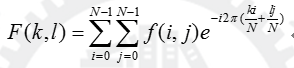其中，f是空间域的值，F是频域的值。

基本数据结构类型：Mat
主要函数：dft() , getOptimalDFTSize(), copyMakeBorder()，magnitude()，normalize()，idft()

---------------------------------------------------------------------------------------------------------------------------------------
voiddft(
InputArraysrc,
OutputArraydst,
intflags=0,
intnonzeroRows=0);            计算DFT的函数

src，存储源图像的Mat
dst，存储图像进行傅立叶变换的结果
flags，转换标识符，默认为0
nonzeroRows，单独处理的行，默认为0

dft()调用方式：
dft(complexI,complexI,DFT_INVERSE);

intgetOptimalDFTSize(int vecsize);            返回傅立叶最优尺寸大小，有利于提高傅立叶运算的速度

getOptimalDFTSize()调用方式：
intm = getOptimalDFTSize(img_in.rows);
intn = getOptimalDFTSize(img_in.cols);

voidcopyMakeBorder(
InputArraysrc,
OutputArraydst,
inttop,
intbottom,
intleft,
intright,
intborderType,
constScalar& value=Scalar() );           作用是扩充图像的边界

src，输入图像
dst，输出图像
top，bottom，left，right，四个方向上需要各扩充的像素宽度
borderType，边界类型，一般选择BORDER_CONSTANT
Scalar，填充的颜色，默认为0

copyMakeBorder()调用方式：
copyMakeBorder(img_in,padded,0,m-img_in.rows,0,n-img_in.cols,BORDER_CONSTANT,Scalar::all(0));

voidmagnitude(
InputArrayx,
InputArrayy,
OutputArraymagnitude);

x，x坐标
y，y坐标
magnitude，根据幅值公式计算得到的公式

magnitude()调用方式：
magnitude(planes,planes,planes);

void normalize(
InputArray src,
OutputArray dst,
double alpha=1,double beta=0, int norm_type=NORM_L2, int dtype=-1, InputArray mask=noArray())

src，输入图像
dst，输出图像
alpha,归一化后最大值，默认为1；beta，归一化后最小值，默认为0
norm_type，归一化类型
dtype，深度类型
mask，可选择的操作掩膜

调用方式：
normalize(magnitudeImage,magnitudeImage,0,1,NORM_MINMAX);

2.进行傅立叶逆变换
源图像转换为频域经过滤波处理后，需要重新转换到空间域上，这时需要用到傅立叶逆变换。
二维图像的傅立叶变换可以用以下的数学公式表达：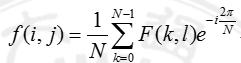主要函数：idft ();

voididft(
InputArraysrc,
OutputArraydst,
intflags=0,
intnonzeroRows=0);            计算IDFT的函数

src，存储源图像的Mat
dst，存储图像进行傅立叶变换的结果
flags，转换标识符，默认为0
nonzeroRows，单独处理的行，默认为0

idft ()调用方式：
idft(complexI,invDFT, DFT_SCALE | DFT_REAL_OUTPUT );

运行结果：
源图像
傅立叶变换图像                          逆变换后图像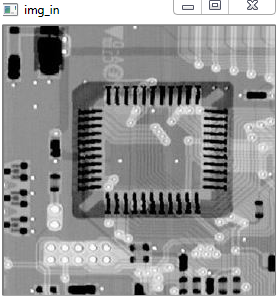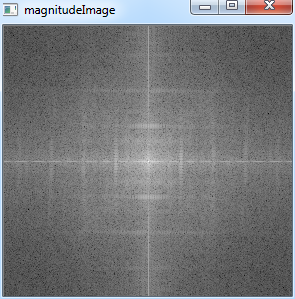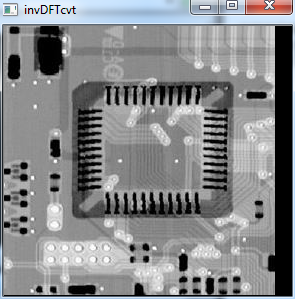程序：
#include <opencv2/core/core.hpp>
#include <opencv2/imgproc/imgproc.hpp>
#include <opencv2/highgui/highgui.hpp>
#include <opencv2/opencv.hpp>
#include <iostream>
using
namespace std;
using
namespace cv;

int main()
{
Mat img_in = imread("origin.JPG",0);
imshow("img_in",img_in);

int m = getOptimalDFTSize(img_in.rows);//计算最佳扩充尺寸
int n = getOptimalDFTSize(img_in.cols);

Mat padded;

copyMakeBorder(img_in,padded,0,m-img_in.rows,0,n-img_in.cols,BORDER_CONSTANT,Scalar::all(0));//扩充图像

Mat planes[]
= {Mat_<float>(padded),Mat::zeros(padded.size(),CV_32F)};//添加维度，用于存储傅立叶变换的结果
Mat complexI;
merge(planes,2,complexI);//合并通道

dft(complexI,complexI,DFT_INVERSE);//离散傅立叶变换

split(complexI,planes);//将存储在complexI的结果分解到planes,planes中
magnitude(planes,planes,planes);//计算复制

Mat magnitudeImage = planes;
magnitudeImage = magnitudeImage
+ Scalar::all(1);
log(magnitudeImage,magnitudeImage);//用对数表示

magnitudeImage = magnitudeImage(Rect(0,0,magnitudeImage.cols
& -2,magnitudeImage.rows
& -2));

int cx = magnitudeImage.cols/2;
int cy = magnitudeImage.rows/2;
Mat q0(magnitudeImage,Rect(0,0,cx,cy));
Mat q1(magnitudeImage,Rect(cx,0,cx,cy));
Mat q2(magnitudeImage,Rect(0,cy,cx,cy));
Mat q3(magnitudeImage,Rect(cx,cy,cx,cy));

Mat tmp;
q0.copyTo(tmp);
q3.copyTo(q0);
tmp.copyTo(q3);

q1.copyTo(tmp);
q2.copyTo(q1);
tmp.copyTo(q2);

normalize(magnitudeImage,magnitudeImage,0,1,NORM_MINMAX);//归一化

imshow("magnitudeImage",magnitudeImage);

Mat invDFT,invDFTcvt;
idft(complexI, invDFT, DFT_SCALE
| DFT_REAL_OUTPUT );//离散傅立叶逆变换
invDFT.convertTo(invDFTcvt, CV_8U);

imshow("invDFTcvt", invDFTcvt);

waitKey(0);
return
0;
}


展开全文OPENCV 图像处理
• 傅里叶变换能将满足一定条件的某个函数表示成三角函数（正弦和/或...在不同的研究领域，傅里叶变换具有多种不同的变体形式，如连续傅里叶变换和离散傅里叶变换。最初傅里叶分析是作为热过程的解析分析的工具被提出的
• STFT（短时傅立叶变换），ISTFT（-短时傅立叶变换），用于音频，麦克风输入 提供25％，50％的重叠STFTCraft.io。 笔记 git clone --recursive https://github.com/kooBH/STFT.git 要构建测试代码，您需要克隆--...
• 快速傅立叶变换和反变换算法实现。源代码和实验报告
• DFT的matlab源代码离散傅里叶变换 DFT快速DFTDFT快速DFT的
• 本代码是一个 Matlab 函数，它提供给定频谱图 STFT(k, l) 的短时傅立叶变换 (ITFT)，其中时间跨列，频率跨行。 函数的输出是： 1) 时域重构信号； 2) 时间向量。 为方便起见，输入输出参数在函数的开头给出 为了...matlab
• matlab开发-有限项序列的离散傅立叶变换。该代码在不使用FFT算法任何预定义的matlab函数的情况下查找idft。
• —————基本公式（傅立叶变换和逆变换）————     ———————线性特性————————   ———————标度变换性质———————— 证明： f(at)= 对称性（了解） 证明： ...
• 快速傅立叶变换（FFT）是一种计算离散傅立叶变换（DFT）及其运算的算法。 傅立叶变换将时间（或空间）转换为频率，反之亦然； FFT可以快速计算出这样的变换。 结果，快速傅里叶变换被广泛应用于工程，科学数学中...
• 无论是傅立叶变换还是小波变换，其实质都是一样的，既：将信号在时间域频率域之间相互转换，从看似复杂的数据中找出一些直观的信息，再对它进行分析。由于信号往往在频域有比在时域更加简单直观的特性，...图像处理 filter fft 工具
• 输入第一个周期序列[1 2 3 1] 输入第二个周期序列[1 2 2 1] 循环卷积输出：11 9 10 12 最终 IDFT 输出：11 9 10 12matlab
• 该程序获取图像（采用最常见的文件格式），并使用快速傅立叶变换方程将其转换为波形，然后将其写入音频文件 (wav)。 如果需要，可以（稍后）将此文件压缩为 mp3。 傅立叶变换允许我们测量时间并转换为频率幅度...
• 图像的均方误差的matlab代码使用傅立叶变换进行图像增强 图像用于各个领域以帮助监视过程，例如指纹评估，卫星监视，医疗诊断，水下区域中的图像。采用图像处理技术作为一种优化方法来有效地处理任务。 傅里叶变换...
• Opencv中的范例中只给出了傅立叶正变换的生成频谱图像的例子，没有给出逆变换和频谱处理的例子，由于最近工作中要用到，就研究了一下用法，理论是几年前在大学学的，现在忘得差不多了，只是简单的回顾了下，所以理论...opencv
• 模拟 2 个已知信号的带通滤波器（它不适用于随机信号）。 - 将信号转换为频域。 - 去除不需要的频率（即 6.5 Hz）。 - 再次将信号转换为... 所有模拟都在没有内置傅立叶传输的情况下完成。 感谢 Mike X Cohen 博士。matlab
• DFT的matlab源代码快速傅立叶变换 Fast Fourier transform (FFT)是一种计算序列的discrete Fourier transform (DFT)或其（ IDFT ）的算法。 让Diecrete Fourier变换（DFT）的形式从O（n ^ {2}）转换为O(nlogn) 。 ...
• 灰度彩色图像的快速傅立叶变换：问题1：对输入的灰度彩色图像进行快速傅立叶变换,显示频谱图; 问题2：进行逆变换,观察结果。 1、问题及说明 （1）对输入的灰度彩色图像进行快速傅立叶变换,显示频谱图； ...
• ## 傅立叶变换详解

千次阅读 2017-08-22 18:55:36
连续傅里叶变换这是将频率域的函数F(ω)表示为时间域的函数f（t）的积分形式连续傅里叶变换的逆变换 (inverse Fourier transform)为：一般可称函数f（t）为原函数，而称函数F(ω)为傅里叶变换的像函数，原函数像...
• 傅立叶变换在时域中获取信号并将其映射到频域，而不会丢失信息。 频域表示是完全相同的信号，形式不同。 傅里叶逆变换 (IDFT) 将信号从频域映射回时域。matlab
• 这句话始终深深的印在我的脑海里，傅立叶变换也就出现了。 想法就是一个非周期函数h(t)，用一个无穷大的周期取重复它自己。 定义 我本想翻译一下，但是感觉没什么意义 维基百科 逆变换之前的傅立叶级数对比...
• Forcot是一种新的一阶曲线（FORC）处理软件，它使用快速傅立叶变换算法来显着加速平滑。 它的目的是为用户提供尽可能简单流畅的体验，以最小的努力来创建打印质量的FORC图。 MATLAB代码需要安装曲线拟合工具箱，...
• * 波浪加载* STFT 具有从 0 到 1 的任何窗口重叠率* MDCT 及其* 常数 Q 变换* 拆分成帧* 发病检测* 音高检测主要读/写属性* s : 信号* 窗口长度（毫秒） * nfft（样本） * 重叠率 (>=0 and <1) * S : stft ...matlab
• 离散傅立叶变换 在大学二年级时，我修了两个学期的信号理论课。 我发现，如果我没有积极地与他们合作，那么就很容易忘记我所学到的概念。 我创建了Python版本的DFT和逆DFT算法，以复习DFT的概念。 dft.py def dft...
• 学习数理化，一大堆外国大Ka命名的定理性质，看了也不知道实质，所以在怀着对大咖无比崇拜的心情的同时，...或者称它们可以通过变换或逆变换得到彼此。因为一般直观的我们都是用时函数来描述信号，所以时函数到频函......